## Saturday, 9 August 2014

### chapter 5 Geometric Theorems

#### Miscellaneous Results

Theorem$28$ Ceva’s theorem
If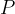$P$is any point inside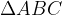$\Delta ABC$ and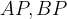$AP,BP$ and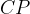$CP$ are produced to meet the sides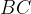$BC$,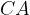$CA$, and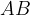$AB$ at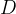$D$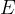$E$ and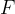$F$ respectively, then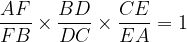$\dfrac{{AF}}{{FB}} \times \dfrac{{BD}}{{DC}} \times \dfrac{{CE}}{{EA}} = 1$.
Conversely, if the above equation holds, then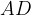$AD$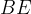$BE$ and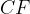$CF$ are concurrent.Theorem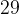$29$ Menelau’s theorem
If$D$$E$ and$F$ are points on$BC$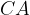$CA$ and$AB$ (suitably produced) respectively of such that$D$$E$ and$F$ are collinear, then$\dfrac{{AF}}{{FB}} \times \dfrac{{BD}}{{DC}} \times \dfrac{{CE}}{{EA}} = 1$.
Conversely, if the above equation holds, then$D$$E$ and$F$ are collinear.Theorem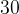$30$ Euler’s theorem
In any triangle, the circumcentre, centroid and orthocentre are collinear and the centroid divides the segment containing the three points in the ratio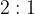$2 : 1$.#### GEOMETRIC THEOREMS

The centroid
The three medians (line from vertex to mid-point of the opposite side) of any triangle are concurrent (meet at the same point). The intersection point is called the centroid of the triangle, usually denoted by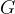$G$. For each median, the length from the vertex to the centroid is twice that from the centroid to the mid-point of the opposite side, i.e.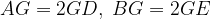$AG = 2GD,\;BG = 2GE$ and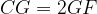$CG = 2GF$.The in-centre
The three angle bisectors (line which divides the angle into two equal halves) of any triangle are concurrent. The intersection point is called the in-centre of the triangle, and it is the centre of the inscribed circle (circle tangent to the three sides of the triangle) of the triangle, usually denoted by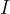$I$.The orthocentre
The three altitudes (from vertex to the opposite side and perpendicular to the opposite side) of any triangle are concurrent. The intersection point is called the orthocentre of the triangle, usually denoted by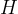$H$.The circumcentre
The three perpendicular bisectors (line from mid-point of each edge perpendicular to the edge) of any triangle are concurrent. The intersection point is called the circumcentre of the triangle, usually denoted by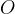$O$, and it is the centre of the circumcircle (circle passing through the three vertices of the triangle). The radius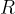$R$ of the circumcircle is called the circumradius of the triangle. By sine law, we have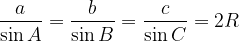$\dfrac{a}{{\sin A}} = \dfrac{b}{{\sin B}} = \dfrac{c}{{\sin C}} = 2R$ where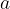$a$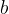$b$ and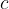$c$are the lengths of the sides opposite to vertices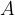$A$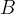$B$ and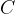$C$.The ex-centre
In any triangle, any two external bisectors (lines that divide the three external angles into two equal halves) and the third angle bisector are concurrent. The three intersection points are called the ex-centres of the triangle and they are the centres of the three ex-circles (circles that are tangent to one of the sides of the triangle and to the extensions of the other two sides).#### Inequalities in Plane Geometry

A lot of facts and theorems of plane geometry are expressed by equalities.
Such is the case of the statements:
“The sum of the angles of a triangle equals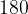$180$ degrees.”
“The square of the hypothenuse of a right angle triangle is equal to the sum of the squares of the other two sides.”
However, inequalities relating various geometric quantities sometimes play even more basic and fundamental role.
We will concentrate on two important inequalities:
(1) The Triangle Inequality: For any three points on the plane the distance between two of the points is no bigger then the sum of the distances from these points to the third one.
In symbols, for any three points$A$$B$$C$ on the plane we have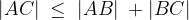$|AC|\; \le \;|AB|\; + |BC|$
Moreover, the equality takes place if and only if the three points are on the same line.
 i.e.,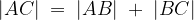$|AC|\; = \;|AB|\; + \;|BC|$ if and only if$A$,$B$,$C$ lie on the same line.
(2) In a triangle, the larger of any two sides is the side opposite to the larger angle.
In symbols, for a triangle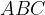$ABC$ we have: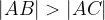$|AB| > |AC|$, if and only if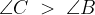$\angle C\; > \;\angle B$